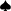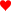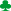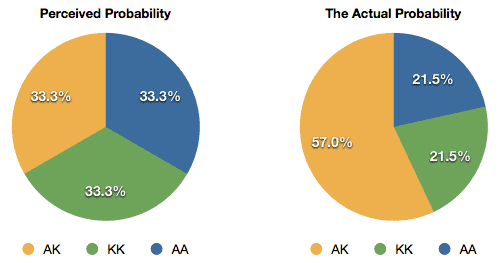# Hand Combinations (Combinatorics)

For a great training video on poker combinatorics, check out this poker combos video."Combinatorics" is a big word for something that isn’t all that difficult to understand. In this article, I will go through the basics of working out hand combinations or "combos" in poker and give a few examples to help show you why it is useful.

Oh, and as you’ve probably noticed, "combinatorics", "hand combinations" and "combos" refer to the same thing in poker. Don’t get confused if I use them interchangeably, which I probably will.

## What is poker combinatorics?

Poker combinatorics involves working out how many different combinations of a hand exists in a certain situation.

For example:

• How many ways can you be dealt AK?
• How many ways can you be dealt 66?
• How combinations of T9 are there on a flop of T32?
• How many straight draw combinations are there on a flop of AT7?

Using combinatorics, you will be able to quickly work these numbers out and use them to help you make better decisions based on the probability of certain hands showing up.

## Poker starting hand combinations basics.

• Any two (e.g. AK or T5) = 16 combinations
• Pairs (e.g. AA or TT) = 6 combinations

If you were take a hand like AK and write down all the possible ways you could be dealt this hand from a deck of cards (e.g. AK, AK, AKetc.), you would find that there are 16 possible combinations.

See all 16 AK hand combinations:Similarly, if you wrote down all the possible combinations of a pocket pair like JJ (e.g. JJ, JJ, JJetc.), you would find that there are just 6 possible combinations.

See all 6 JJ pocket pair hand combinations:So as you can see from these basic starting hand combinations in poker, you’re almost 3 times as likely to be dealt a non-paired hand like AK than a paired hand. That’s pretty interesting in itself, but you can do a lot more than this…

### Note: two extra starting hand combinations.

As mentioned above, there are 16 combinations of any two non-paired cards. Therefore, this includes the suited and non-suited combinations.

Here are 2 extra stats that give you the total combinations of any two suited and any two unsuited cards specifically.

• Any two (e.g. AK or 67 suited or unsuited) = 16 combinations
• Any two suited (AKs) = 4 combinations
• Any two unsuited (AKo) = 12 combinations
• Pairs (e.g. AA or TT) = 6 combinations

You won’t use these extra starting hand combinations nearly as much as the first two, but I thought I would include them here for your interest anyway.

It’s easy to work out how there are only 4 suited combinations of any two cards, as there are only 4 suits in the deck. If you then take these 4 suited hands away from the total of 16 "any two" hand combinations (which include both the suited and unsuited hands), you are left with the 12 unsuited hand combinations. Easy.

Fact: There are 1,326 combinations of starting hands in Texas Hold’em in total.

## Working out hand combinations using "known" cards.

Let’s say we hold KQ on a flop of KT4 (suits do not matter). How many possible combinations of AK and TT are out there that our opponent could hold?

### Unpaired hands (e.g. AK).How to work out the total number of hand combinations for an unpaired hand like AK, JT, or Q3.

Method: Multiply the numbers of available cards for each of the two cards.
Word equation: (1st card available cards) x (2nd card available cards) = total combinations

#### Example.

If we hold KQ on a KT4 flop, how many possible combinations of AK are there?

There are 4 Aces and 2 Kings (4 minus the 1 on the flop and minus the 1 in our hand) available in the deck.

``````C = A1 * A2
C = 4 x 2
C = 8
``````

C = 8, so there are 8 possible combinations of AK if we hold KQ on a flop of KT4.

### Paired hands (e.g. TT).How to work out the total number of hand combinations for an paired hand like AA, JJ, or 44.

Method: Multiply the number of available cards by the number of available cards minus 1, then divide by two.
Word equation: [(available cards) x (available cards - 1)] / 2 = total combinations

#### Example.

How many combinations of TT are there on a KT4 flop?

Well, on a flop of KT4 here are 3 Tens left in the deck, so…

``````C = [(A) * (A-1)] / 2
C = [(3) * (3-1)] / 2
C = [3 * 2] / 2
C = 3
``````

C = 3, which means there are 3 possible combinations of TT.

### Thoughts on working out hand combinations.

Working out the number of possible combinations of unpaired hands is easy enough; just multiply the two numbers of available cards.

Working out the combinations for paired hands looks awkward at first, but it’s not that tricky when you actually try it out. Just find the number of available cards, take 1 away from that number, multiply those two numbers together then half it.

Note: You’ll also notice that this method works for working out the preflop starting hand combinations mentioned earlier on. For example, if you’re working out the number of AK combinations as a starting hand, there are 4 Aces and 4 Kings available, so 4 x 4 = 16 AK combinations.

## Why is combinatorics useful?

Because by working out hand combinations, you can find out more useful information about a player’s range.

For example, let’s say that an opponents 3betting range is roughly 2%. This means that they are only ever 3betting AA, KK and AK. That’s a very tight range indeed.

Now, just looking at this range of hands you might think that whenever this player 3bets, they are more likely to have a big pocket pair. After all, both AA and KK are in his range, compared to the single unpaired hand of AK. So without considering combinatorics for this 2% range, you might think that the probability break-up of each hand looks like this:

• AA = 33%
• KK = 33%
• AK = 33%

…with the two big pairs making up the majority of this 2% 3betting range (roughly 66% in total).

However, let’s look at these hands by comparing the total combinations for each hand:

• AA = 6 combinations (21.5%)
• KK = 6 combinations (21.5%)
• AK = 16 combinations (57%)There are more AK hands in a range of [AA, KK, AK] than there are AA and KK hands combined.

So out of 28 possible combinations made up from AA, KK and AK, 16 of them come from AK. This means that when our opponent 3bets, the majority of the time he is holding AK and not a big pocket pair.

Now obviously if you’re holding a hand like 75o this is hardly comforting. However, the point is that it’s useful to realise that the probabilities of certain types of hands in a range will vary. Just because a player either has AA or AK, it doesn’t mean that they’re both equally probable holdings - they will actually be holding AK more often than not.

Analogy: If a fruit bowl contains 100 oranges, 1 apple, 1 pear and 1 grape, there is a decent range of fruit (the "hands"). However, the the fruits are heavily weighted toward oranges, so there is a greater chance of randomly selecting an orange from the bowl than any of the 3 other possible fruits ("AK" in the example above).

This same method applies when you’re trying to work out the probabilities of a range of possible made hands on the flop by looking at the number of hand combinations. For example, if your opponent could have either a straight draw or a set, which of the two is more likely?

## Poker combinatorics example hand.

You have 66 on a board of AJ682. The pot is \$12 and you bet \$10. Your opponent moves all in for \$60, which means you have to call \$50 to win a pot of \$82.

You are confident that your opponent either has a set or two pair with an Ace (i.e. AJ, A8, A6 or A2). Don’t worry about how you know this or why you’re in this situation, you just are.

According to pot odds, you need to have at least a 38% chance of having the best hand to call. You can now use combinatorics / hand combinations here to help you decide whether or not to call.

### Poker combinatorics example hand solution.

First of all, let’s split our opponent’s hands in to hands you beat and hands you don’t beat, working out the number of hand combinations for each.

``````Hands you beat.

AJ = 3 x 3 = 9 combinations
A8 = 3 x 3 = 9 combinations
A6 = 3 x 1 = 3 combinations
A2 = 3 x 3 = 9 combinations
22 = (3 x 2) / 2 = 3 combinations
``````
``````Hands you don’t beat.

AA = (3 x 2) / 2 = 3 combinations
JJ = (3 x 2) / 2 = 3 combinations
88 = (3 x 2) / 2 = 3 combinations
``````

``````Total combinations = 42

Combinations you beat = 33 (79%)
Combinations you don’t beat = 9 (21%)
``````

Seeing as you have the best hand 79% of the time (or 79% "equity") and the pot odds indicate that you only need to have the best hand 38% of the time, it makes it +EV to call.

So whereas you might have initially thought that the number of hands we beat compared to the number of hands we didn’t beat was close to 50/50 (making it likely -EV to call), after looking at the hand combinations we can see that it is actually much closer to 80/20, making calling a profitable play.

Being able to assign a range to your opponent is good, but understanding the different likelihoods of the hands within that range is better.

## Poker combinatorics conclusion.

Working out hand combinations in poker is simple:

• Unpaired hands: Multiply the number of available cards. (e.g. AK on an AT2 flop = [3 x 4] = 12 AK combinations).
• Paired hands: Find the number of available cards. Take 1 away from that number, multiply those two numbers together and divide by 2. (e.g. TT on a AT2 flop = [3 x 2] / 2 = 3 TT combinations).

By working out hand combinations you can gain a much better understanding about opponent’s hand ranges. If you only ever deal in ranges and ignore hand combinations, you are missing out on useful information.

It’s unrealistic to think that you’re going to work out all these hand combinations on the fly whilst you’re sat at the table. However, a lot of value comes from simply familiarizing yourself with the varying probabilities of different types of hands for future reference.

For example, after a while you’ll start to realise that straight draws are a lot more common than you think, and that flush draws are far less common than you think. Insights like these will help you when you’re faced with similar decisions in the future.

The next time you’re doing some post session analysis, spend some time thinking about combinatorics and noting down what you find.

Hand combinations in poker all stem from statistics. So if you’re interested in finding out more about the math side of things, here are a few links that I found helpful:

If you’re more interested in finding out more about combinations in poker only, here are a few interesting reads:

Go back to the awesome Texas Hold'em Strategy.